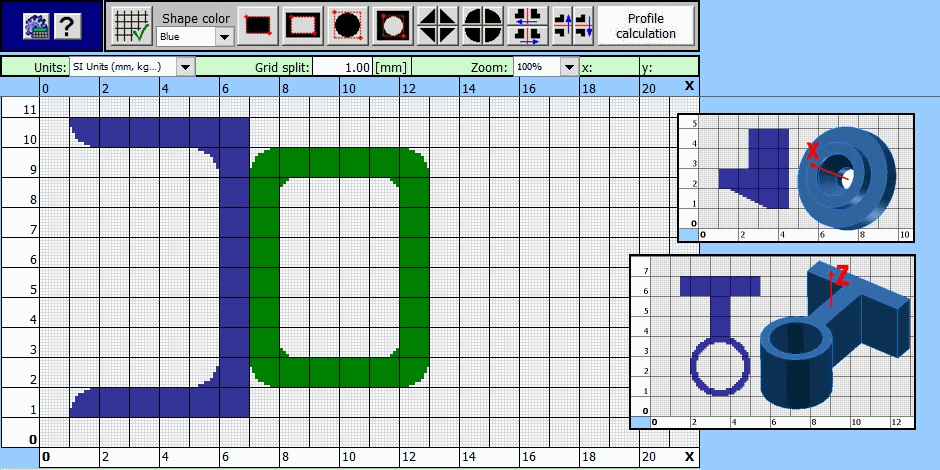Products /

# Profiles, Area Characteristics, Moment of Inertia

The calculation solves area characteristics of common profiles and mass characteristics of solids created by drawing or rotation of the profile.

The application enables:

• Creation of a profile in a simple graphic editor.
• Calculation of area characteristics for main axes passing through the centre of gravity (moment of inertia, radius of gyration, bending modulus...).
• Calculation of area characteristics (Ix, Sx) for randomly turned axes passing through the centre of gravity, including a graph.
• Calculation of characteristics for the turned axes which pass through a random point.
• Calculation of volume and mass characteristics (V, m, Im) of solids created by drawing or rotation of the profile.